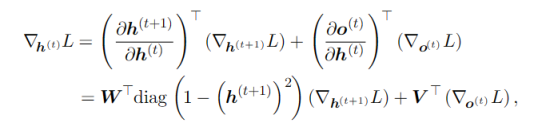Instantly share code, notes, and snippets.

# karpathy/min-char-rnn.py

Last active December 3, 2023 18:09
Star You must be signed in to star a gist
Minimal character-level language model with a Vanilla Recurrent Neural Network, in Python/numpy
This file contains bidirectional Unicode text that may be interpreted or compiled differently than what appears below. To review, open the file in an editor that reveals hidden Unicode characters. Learn more about bidirectional Unicode characters

### anantinfinity9796 commented Jun 20, 2022

Hi, can anyone explain the line where we pass in the gradient through the softmax function " dy [targets[t]] -=1 ". Why are we doing this operation ??

### sashakttripathi commented Nov 10, 2022

To anyone finding the code hard to understand, I provide detailed explanations here: https://mkffl.github.io/ Hope it helps

Good explanation for second part of lossFunction.

### pjoer commented Jan 31, 2023 • edited

Could someone explain how to use this? I can't generate text. It is running though.

### ijkilchenko commented Jan 31, 2023

@pjoer, do you have an input.txt file (really any text file) in the same directory of this file which you should be running like python min-char-rnn.py

It seems the above was written with python2 in mind, but I just updated a few lines and got it working on Python 3.9.6: https://gist.github.com/ijkilchenko/84be862a5e18240c59b4505177c9c34c

Good luck!

### pursuemoon commented Sep 24, 2023 • edited

I have a problem in the code of line 58: dhnext = np.dot(Whh.T, dhraw). Could anyone tell me what it means?

The expression for forward propagation is:

\begin{align} z_t & = W_{hh} \cdot h_{t-1} + W_{xh} \cdot x_t + b_h \tag{1}\\ h_t & = Tanh(z_t) \tag{2}\\ y_t & = W_{hy} \cdot h_t + b_y \tag{3}\\ o_t & = Softmax(y_t) \tag{4}\\ \end{align}

Here is the gradients expression of weights that I derived:

\begin{align} \frac{\partial L}{\partial W_{hy}} & = \frac{\partial L}{\partial y_t} \otimes {h_t}^T \tag{a}\\ \frac{\partial L}{\partial W_{hh}} & = {\color{red} {W_{hy}}^T \cdot \frac{\partial L}{\partial y_t} \odot Tanh'(z_t)} \otimes {h_{t-1}}^T \tag{b}\\ \frac{\partial L}{\partial W_{xh}} & = {\color{red} {W_{hy}}^T \cdot \frac{\partial L}{\partial y_t} \odot Tanh'(z_t)} \otimes x_t^T \tag{c} \end{align}

It is easy to see that the red part is what dhraw represents in the code. And we can get dWxh and dWhh from formula (b) and formula (c) without dhnext. So what does dhnext mean?

### logeek404 commented Oct 24, 2023

Stupid question : In which lines does the vanishing gradient problem manifest itself ?

It uses adaGrad optimization in the last few lines. Hence the gradient is always divided by the accumulate sum of each gradients' scaler .
mem += dparam*dparam
param += -learning_rate * dparam / np.sqrt(mem+1e-8)

However , I find that the mem and param are all local variables in the loop. Don't know if the implementation of adaGrad is correct.

### logeek404 commented Oct 24, 2023

I have a problem in the code of line 58: dhnext = np.dot(Whh.T, dhraw). Could anyone tell me what it means?

The expression for forward propagation is:

(1)zt=Whh⋅ht−1+Wxh⋅xt+bh(2)ht=Tanh(zt)(3)yt=Why⋅ht+by(4)ot=Softmax(yt)

Here is the gradients expression of weights that I derived:

(a)∂L∂Whh=∂L∂yt⊗htT(b)∂L∂Whh=WhyT⋅∂L∂yt⊙Tanh′(zt)⊗ht−1T(c)∂L∂Wxh=WhyT⋅∂L∂yt⊙Tanh′(zt)⊗xtT

It is easy to see that the red part is what dhraw represents in the code. And we can get dWxh and dWhh from formula (b) and formula (c) without dhnext. So what does dhnext mean?Since dh means the gradient of loss wrt the hidden state, there are two ways the gradients flow(back propogation). From the equation and rnn structure we learn that the hidden state feeds forward to a output and next hidden state. The dhnext represents the gradient update for current state from the next hidden state. Note that dhnext is zero at first iteration because for the last-layer (unrolled) of rnn , there 's not a gradient update flow from the next hidden state.

### pursuemoon commented Oct 27, 2023

I have a problem in the code of line 58: dhnext = np.dot(Whh.T, dhraw). Could anyone tell me what it means?
The expression for forward propagation is:
(1)zt=Whh⋅ht−1+Wxh⋅xt+bh(2)ht=Tanh(zt)(3)yt=Why⋅ht+by(4)ot=Softmax(yt)
Here is the gradients expression of weights that I derived:
(a)∂L∂Whh=∂L∂yt⊗htT(b)∂L∂Whh=WhyT⋅∂L∂yt⊙Tanh′(zt)⊗ht−1T(c)∂L∂Wxh=WhyT⋅∂L∂yt⊙Tanh′(zt)⊗xtT
It is easy to see that the red part is what dhraw represents in the code. And we can get dWxh and dWhh from formula (b) and formula (c) without dhnext. So what does dhnext mean?Since dh means the gradient of loss wrt the hidden state, there are two ways the gradients flow(back propogation). From the equation and rnn structure we learn that the hidden state feeds forward to a output and next hidden state. The dhnext represents the gradient update for current state from the next hidden state. Note that dhnext is zero at first iteration because for the last-layer (unrolled) of rnn , there 's not a gradient update flow from the next hidden state. Hope this will help you .

Thank you so much for your replying. I missed the partial derivative wrt the next hidden state.